Published: 30 June 2016

# Multi-objective optimization design for a battery pack of electric vehicle with surrogate models

Cheng Lin1
Fengling Gao2
Wenwei Wang3
Xiaokai Chen4
1, 2, 3, 4National Engineering Laboratory for Electric Vehicles, School of Mechanical Engineering, Beijing Institute of Technology, Beijing 100081, China
1, 2, 3, 4Collaborative Innovation Center of Electric Vehicles in Beijing, Beijing Institute of Technology, Beijing 100081, China
Corresponding Author:
Fengling Gao
Views 2055

#### Abstract

In this investigation, a systematic surrogate-based optimization design framework for a battery pack is presented. An air-cooling battery pack equipped on electric vehicles is first designed. Finite element analysis (FEA) results of the baseline design show that global maximum stresses under $x$-axis and $y$-axis transient acceleration shock condition are both above the tensile limit of material. Selecting the panel and beam thickness of battery pack as design variables, with global maximum stress constraints in shock cases, a multi-objective optimization problem is implemented using metamodel technique and multi-objective particle-swarm-optimization (MOPSO) algorithm to simultaneously minimize the total mass and maximize the restrained basic frequency. It is found that 2nd order polynomial response surface (PRS), 3rd order PRS and radial basis function (RBF) are the most accurate and appropriate metamodels for restrained basic frequency, global maximum stresses under x-axis and y-axis shock conditions respectively. Results demonstrate that all the optimal solutions in Pareto Frontier have heavier weight and lower frequency compared with baseline design due to the restriction of global maximum stress response. Finally, two optimal schemes, “Knee Point” and “lightest weight”, satisfied both of the stress constraint conditions, show great consistency with FEA results and can be selected as alternative improved schemes.

## 1. Introduction

Under the premise of meeting structure design requirements, battery pack assembly should be as light as possible for energy-saving and mileage elongation consideration. Therefore, given a designed battery pack with topology and shape fixed components, to satisfy the above-mentioned demands, it must be of a multi-objective and multi-constraint size optimization problem. Handling structure optimization problem with many of FEA in objective and constraint function evaluations employing either gradient based or gradient free optimization techniques are quite computationally expensive, particularly terrible with increasing number of variables . In order to improve the computational efficiency in such design problems, the concept of “metamodel” or “surrogate model” approximating the physical model has been introduced. The metamodel is constructed in light of a sufficient number of sampling points, typically determined through experiments. Selecting a Design of Experiment (DoE) method for data generation, choosing a model to represent the data, fitting the model and finally validating its accuracy are the four basic steps in metamodeling. Polynomial response surface (PRS) , Kriging (KRG)  and radial basis function (RBF)  are widely applied as surrogate models to substitute time-consuming FEA in structure optimization. It is obviously that different type of surrogate model is applied for modeling varied structural mechanics response depending on specific research object [14, 18-26]. For this reason, a study about selecting the most accurate and appropriate metamodel for predicting different structural mechanics response in battery pack optimization design is necessary. Particle swarm optimization (PSO), which is a type of swarm intelligent optimization algorithm, begins with a set of initial random solutions to obtain an optimal solution by repeated iterations. Multi-objective PSO (MOPSO) is the improved algorithm of PSO . For having relatively fast convergence and attaining well-distributed Pareto frontier, surrogate model based MOPSO has been an effective optimizing measure in structural optimization [14, 22-25]. However, there is almost no report about battery box optimization with surrogate model based MOPSO.

In this research work, a systematic and elaborate surrogate-based optimization design methodology for an air-cooling battery pack is presented. After implementing FEA of the baseline design, it is found that the global maximum stresses under its $x$-axis and $y$-axis transient acceleration shock condition are both above the tensile limit of material. A multi-objective size optimization problem is conducted using surrogate model technique and multi-objective particle swarm optimization (MOPSO) algorithm to simultaneously minimize the total mass and maximize the restrained basic frequency with the global maximum stress constraint in transient acceleration shock cases. To efficiently approximate the responses of interest, several widely-used surrogate modeling techniques such as KRG, PRS, and RBF are compared with each other. The optimal schemes derived will demonstrate their better structure performance than the baseline design.

## 2. Methodology

### 2.1. Optimal Latin hypercube sampling for design of experiment

In engineering design, direct coupling of optimization algorithm with simulation model may not be efficient since iterative calculation and sensitivity analysis usually require enormously computational efforts. As an alternative, the metamodels or surrogate modeling techniques have been proven as an effective substitute of costly simulation analysis for optimization [14, 18-25]. In practice, surrogate modeling technique starts with the sampling data at some training points. Design of experiment (DoE) is an approach to address how to select training points effectively and appropriately. In this paper, the optimal Latin Hypercube sampling (OLHS) approach is employed to generate initial training points . In order to find a set of training points with the highest uniformity, ${L}_{P}$ discrepancy is used as the criterion to gauge the uniformity in this study. Presume that there are $N$ factors of interest over a standard domain ${C}^{N}$. The goal is to choose a set of training points ${x}_{S}=\left\{{x}_{1},{x}_{2},\dots ,{x}_{{n}_{S}}\right\}$ such that these points are uniformly scattered on ${C}^{N}$. To seek a set of ${x}_{S}$ that maximizes the uniformity over all possible ${n}_{S}$ points on ${C}^{N}$, the centered ${L}_{2}$ discrepancy criterion is used in our study, given as :

1
$C{L}_{2}\left({x}_{S}\right)=\left(\frac{13}{12}\right)N-\frac{2}{{n}_{S}}\sum _{k=1}^{{n}_{S}}\prod _{l=1}^{N}\left[1+\frac{1}{2}\left|{x}_{k}^{l}-0.5\right|-\frac{1}{2}{\left|{x}_{k}^{l}-0.5\right|}^{2}\right]$
$+\frac{1}{{n}_{S}^{2}}\sum _{k=1}^{{n}_{S}}\sum _{j=1}^{{n}_{S}}\prod _{i=1}^{N}\left[1+\frac{1}{2}\left|{x}_{k}^{i}-0.5\right|-\frac{1}{2}\left|{x}_{j}^{i}-0.5\right|-\frac{1}{2}\left|{x}_{j}^{i}-{x}_{k}^{i}\right|\right].$

### 2.2. Metamodels in multi-objective sequential optimization

In the optimization process, the surrogate models, specifically, polynomial response surface (PRS) , Kriging (KRG) , radial basis function (RBF)  and etc, have been widely adopted as effective tools to approximate structural performances, and can largely reduce the number of costly finite element analysis (FEA) runs to improve the optimization efficiency and feasibility. Surrogate model technique starts with training data at sampling points as above-mentioned, and then constructs functional relationship between design variables and responses so as to predict the values at any points in the design space.

PRS model is one of the simplest and most popular surrogate models. As an effective alternative to FEA, PRS model has been widely adopted in design optimization [14, 23, 30]. Mathematically PRS metamodels from the first to the fourth order can be, respectively, written as:

2
$y=a+\sum _{i=1}^{t}{b}_{i}{x}_{i},$
$y=a+\sum _{i=1}^{t}{b}_{i}{x}_{i}+\sum _{i=1}^{t}{c}_{ii}{x}_{i}^{2}+\sum _{i=1}^{t}\sum _{i
$y=a+\sum _{i=1}^{t}{b}_{i}{x}_{i}+\sum _{i=1}^{t}{c}_{ii}{x}_{i}^{2}+\sum _{i=1}^{t}\sum _{i
$y=a+\sum _{i=1}^{t}{b}_{i}{x}_{i}+\sum _{i=1}^{t}{c}_{ii}{x}_{i}^{2}+\sum _{i=1}^{t}\sum _{i

where ${x}_{i}$ ($i=$ 1, 2,…, $t$) denotes the design variables determining response $y$; based on the least square method, estimated regression coefficients, namely, $a$, ${b}_{i}$, ${c}_{ii}$, ${e}_{ii}$, ${f}_{ii}$ and ${d}_{ij}$ can be obtained. ${x}_{i}{x}_{j}$ is the cross term that represents two-parameter interaction and the higher-order terms stand for the nonlinearity characteristic. In general, the order of PRS is no higher than 4.

The KRG model was originally developed for mining and geostatistical applications involving spatially and temporally correlated data. The stochastic process function $y\left(x\right)$ is used to describe the deterministic response of a system, which contains a regression model and a stochastic error :

3
$y\left(x\right)={f\left(x\right)}^{T}+z\left(x\right),$

where ${\beta =\left[{\beta }_{1},{\beta }_{2},\dots ,{\beta }_{p}\right]}^{T}\text{,}\text{}{f\left(x\right)=\left[{f}_{1}\left(x\right),{f}_{2}\left(x\right),\dots ,{f}_{p}\left(x\right)\right]}^{T}\text{,}$ are the column vectors of regression parameters and basis functions, respectively; p represents the number of basis functions; $z\left(x\right)$ denotes a stochastic parameter with zero mean and variance as ${\sigma }^{2}$. The covariance matrix of $z\left(x\right)$ is shown as:

4
$\mathrm{C}\mathrm{o}\mathrm{v}\left[z\left({x}_{i}\right),z\left({x}_{j}\right)\right]={\sigma }^{2}R\left[R\left({x}_{i},{x}_{j}\right)\right],$

where $R$ is a correlation matrix defined by Gaussian correlation function $R\left({x}_{i},{x}_{j}\right)$ as follows:

5
$R\left({x}_{i},{x}_{j}\right)=\mathrm{exp}\left[-\sum _{k=1}^{{n}_{dv}}{{\theta }_{k}\left|{x}_{i}^{k}-{x}_{j}^{k}\right|}^{2}\right],$

here ${n}_{dv}$ is the number of design variables, ${\theta }_{k}$ is the unknown correlation parameter used to fit the model, and ${x}_{i}^{k}$ and ${x}_{j}^{k}$ are the $k$th component of training points ${x}_{i}$ and ${x}_{j}$, respectively.

Then, $\stackrel{^}{y}\left(x\right)$ is employed as predicted estimate of response $y\left(x\right)$, expressed as:

6
$\stackrel{^}{y}\left(x\right)={f\left(x\right)}^{T}\stackrel{^}{\beta }+{r}^{T}\left(x\right){R}^{-1}\left({y}_{s}-F\stackrel{^}{\beta }\right),$

where ${{y}_{s}=\left[y\left({x}_{1}\right),y\left({x}_{2}\right),\dots ,y\left({x}_{{n}_{S}}\right)\right]}^{T}$ is the response vector of the ${\mathrm{n}}_{\mathrm{S}}$ training points ${x}_{s}=\left\{{x}_{1},{x}_{2},\dots ,{x}_{{n}_{S}}\right\}\text{,}$ and ${F=\left[f\left({x}_{1}\right),f\left({x}_{2}\right),\dots ,f\left({x}_{{n}_{S}}\right)\right]}^{T}$ is a ${n}_{S}×p$ matrix. ${{r}^{T}\left(x\right)=\left[R\left(x,{x}_{1}\right),R\left(x,{x}_{2}\right),\dots ,R\left(x,{x}_{{n}_{S}}\right)\right]}^{T}$ is a correction vector that implies how close between training points and untrained points. $\stackrel{^}{\beta }$ is the general least square estimator formulated as follows:

7
$\stackrel{^}{\beta }={\left({F}^{T}{R}^{-1}F\right)}^{-1}{F}^{T}{R}^{-1}{y}_{s}.$

The estimate to the variance of training data from the global model is:

8
${\stackrel{^}{\sigma }}^{2}=\frac{{\left({y}_{s}-F\stackrel{^}{\beta }\right)}^{T}{R}^{-1}\left({y}_{s}-F\stackrel{^}{\beta }\right)}{{n}_{S}}.$

For calculating ${\theta }_{k}$ in Eq. (5), the maximum likelihood estimates can be used by solving the following maximization problem over the interval ${\theta }_{k}>0$, as:

9
$\mathrm{max}\left(-\frac{{n}_{S}\mathrm{ln}\left({\stackrel{^}{\sigma }}^{2}\right)+\mathrm{l}\mathrm{n}\left|R\right|}{2}\right),$

where both ${\stackrel{^}{\sigma }}^{2}$ and $\left|R\right|$ are the functions of ${\theta }_{k}$.

RBF model exhibits superior prediction ability and ensures good accuracy in highly nonlinear responses problem . The RBF model consists of the linear combination of basis function, and the basis function in RBF is given as:

10
${\phi }_{i}\left(x\right)=\phi \left(‖x-{\mu }^{i}‖\right)=\phi \left(\xi \right),$

where $\xi =‖x-{\mu }^{i}‖$, is an independent variable representing the Euclidean distance between the design point $x$ and the observed input ${\mu }^{i}$. The RBF model is given as:

11
$\stackrel{^}{y}=\sum _{i}{a}_{i}\phi \left(‖x-{\mu }^{i}‖\right)+\theta .$

The accuracy of surrogate models should be assessed after they are established by adding extraneous confirmation sample points. Three metrics adopted herein are R-square, relative average absolute error (RAAE), and relative maximum absolute error (RMAE) , respectively, as:

12
${R}^{2}=1-\frac{{\sum _{i=1}^{q}\left({y}_{i}-{\stackrel{^}{y}}_{i}\right)}^{2}}{{\sum _{i=1}^{q}\left({y}_{i}-\stackrel{-}{y}\right)}^{2}},$
$RAAE=\frac{\sum _{i=1}^{q}\left|{y}_{i}-{\stackrel{^}{y}}_{i}\right|}{\sum _{i=1}^{q}\left|{y}_{i}-\stackrel{-}{y}\right|},$
$RMAE=\frac{\mathrm{m}\mathrm{a}\mathrm{x}\left\{\left|{y}_{i}-{\stackrel{^}{y}}_{1}\right|,\dots ,\left|{y}_{i}-{\stackrel{^}{y}}_{q}\right|\right\}}{\sum _{i=1}^{q}\left({y}_{i}-\stackrel{-}{y}\right)/q},$

where ${y}_{i}$ and ${\stackrel{^}{y}}_{i}$ indicate the exact function value and the corresponding surrogate value at confirmation point $i$, respectively. $\stackrel{-}{y}$ is the mean of ${y}_{i}$, $q$ is the number of the confirmation points. Generally, a larger value of R-square and a smaller value of RAAE inferring a higher accuracy for overall performance in the design space are preferred. On the other hand, a larger RMAE value denotes less accuracy in one region of the design space even though a very good global measurement can be given by R-square and RAAE. In optimization applications, the first two metrics are usually paid more attention for the global behavior concerning.

### 2.3. Multi-objective particle swarm optimization (MOPSO) algorithm

Particle swarm optimization (PSO), is a relatively new heuristic and derivative-free global optimum algorithm inspired by the choreography of a bird flock . It starts with a set of initial random solutions to obtain an optimal solution by repeated iterations. The multi-objective particle swarm optimization (MOPSO)  is an extended version to PSO, which has gained considerable attention for its capacity of fast convergence and obtaining well-distributed Pareto frontier. To maintain the distribution uniformity of solutions, MOPSO uses an external archive to save the non-dominated solutions during the search procedure and clips the non-dominated solutions by a crowded degree algorithm. Finally, MOPSO selects the elite individual from the sparse regions in the external archive as the global optimal solution.

## 3. Design optimization of battery pack

### 3.1. Finite element modeling

As a critical and independent part assembled to carry and protect Lithium-ion power battery set, especially considering of the complicated driving condition of electric vehicle, the battery pack requires high mechanics performance to carry out its functions. Fig. 1(a) and (b) display the global geometric model and inner structure model of an air-cooling battery pack using the commercial software Catia.

Based on the FEA code Hypermesh, this battery pack, composed of panels and beams, is modeled mainly with PSHELL shell elements with their material property of Q235 steel assigned linear elastic isotropic material model MAT1. For connectivity, REB2 and CWELD elements are used to model weld spots, as well as 1D PBAR element for the representative of battery connecting bars. For lack of detailed information about battery material and mechanics property, like other studies [4, 6-8], some simplified treatment measurements are employed in this research to assure the mechanical effect of battery on the whole pack structure. Taking account of cuboid geometry shape feature, battery sets are substituted by PSHELL elements with appropriate thickness and density. A much lower elastic modulus of the battery compared to steel is adopted here for neglecting its own mechanical response in FEA process. The entire FEA model has 117837 elements with the total mass of 0.3211 ton, which is shown in Fig. 2.

Fig. 1Global geometric model and inner structure model of the battery pack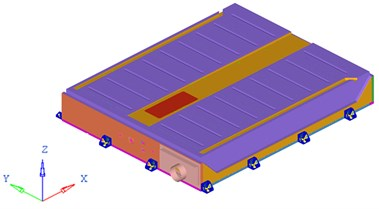a) Global geometric model of the battery pack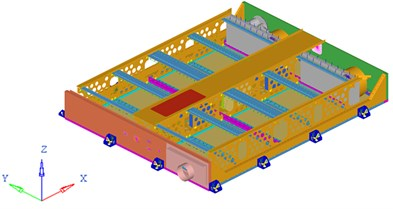b) Inner structure model of the battery pack

Fig. 2FEA model of the battery pack and its inner battery sets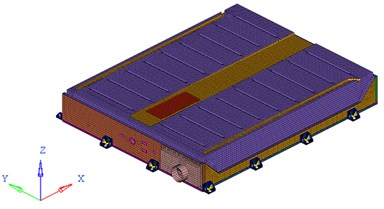a) FEA model of the battery pack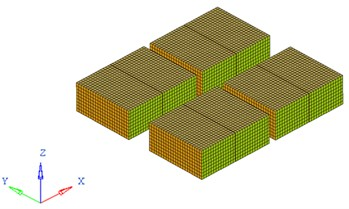b) FEA model of the inner battery sets

Similar to [4, 6-8], as an important load-condition in structure analysis and optimization for battery pack, restrained modal analysis is studied in this work. As the boundary condition shown in Fig. 3, 6 freedoms of each connecting position between battery pack bottom plate and the vehicle body are constrained.

Fig. 3Boundary condition of battery pack FEA model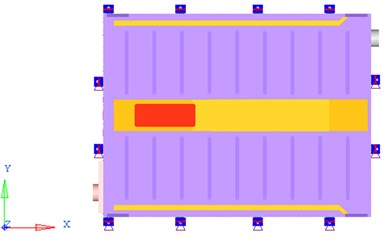A higher basic frequency (1st order restrained modal frequency) of battery pack can make it avoid the resonance with the vehicle , which is concerned most in this work. The first 20 order restrained frequencies and corresponding positions of vibration are illustrated in Table 1. Fig. 4 demonstrates the vibration mode of the first order frequency.

Besides, limited driving conditions of vehicles, such as frontal-collision and side-collision happened in a very short time, would cause super large acceleration acting on all the components . For the sake of battery safety, the battery pack should not happen to crack under such transient shock, which means stress in the battery pack should not exceed the material tensile limit. Consequently, in this research, according to the regulations described in Chinese Automobile industry standard – General Requirement of Traction Battery Enclosure for Electric Vehicles (QC/T 989-2014), transient shock response of the battery pack under 40 g acceleration acted along its $x$-axis and $y$-axis in 6 ms, is concerned . The loading process of acceleration excitation is shown in Fig. 5.

Table 1The first 20 order restrained frequencies and corresponding positions of vibration of the battery pack

 Order Frequency / Hz Position 1 38 Entirety 2 43 Entirety 3 50 Bottom plate 4 50 Bottom plate 5 50 Bottom plate 6 51 Bottom plate 7 51 Bottom plate 8 52 Bottom plate 9 54 Bottom plate 10 55 Bottom plate 11 56 Bottom plate 12 59 Entirety 13 67 Bellows 14 71 Cover 15 72 Cover 16 73 Cover 17 76 Entirety 18 76 Entirety 19 76 Bottom plate 20 77 Bottom plate

Fig. 4The vibration mode of first order frequency of the battery pack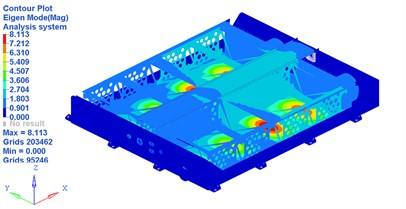a) Main view of the vibration mode of first order frequency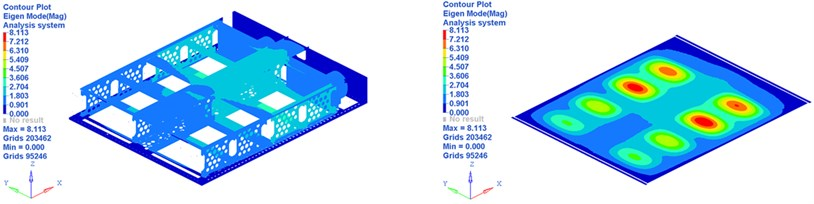b) Inner view of the vibration mode of first order frequency

Through the FEA results displayed in Fig. 6, it is easy to find that the maximum von Mises stresses in battery pack $x$-axis and $y$-axis transient shock cases are both above 380 MPa, the tensile limit of steel. Therefore, security risk exists in this battery pack, and a redesign is necessary.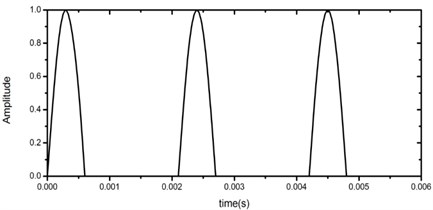Fig. 6FEA results of x-axis and y-axis transient shock on the battery pack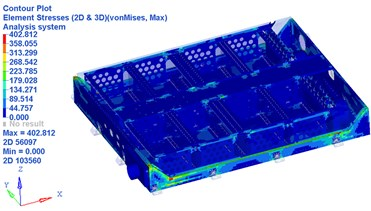a) FEA results of $x$-axis transient shock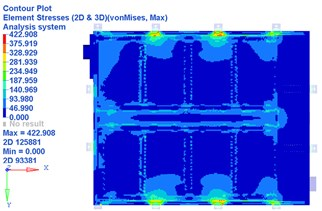b) FEA results of $y$-axis transient shock

### 3.2. Definition of optimization problem

An excellent battery pack structure design should meet all of the mechanical performance requirements, in conjunction with lighter weight for energy-saving and mileage elongation consideration. Of all the mechanical properties, its first order restrained natural frequency ought to be as high as possible to avoid the resonance with the vehicle. Therefore, the basic frequency is maximized and at the same time the structural mass is minimized, whilst maintaining certain levels of other transient shock response indices along its $x$-axis and $y$-axis as mentioned. In this study, thicknesses of seven panels and one beam set in Fig. 7 are selected as the design variables whose ranges are all from 0.5 mm to 1.5 mm. The detailed information of these design variables are depicted in Table 2.

Fig. 7Illustration of design variables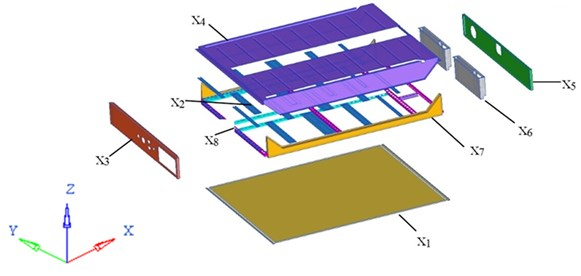The multi-objective size optimization problem for battery pack is thus specifically formulated as follows:

13
$\mathrm{M}\mathrm{i}\mathrm{n}\mathrm{i}\mathrm{m}\mathrm{i}\mathrm{z}\mathrm{e}\left[{F}_{m}\left(x\right),-{F}_{f}\left(x\right)\right],$
$\mathrm{S}\mathrm{u}\mathrm{b}\mathrm{j}\mathrm{e}\mathrm{c}\mathrm{t}\mathrm{}\mathrm{t}\mathrm{o}:$
$\left\{\begin{array}{l}0.5\mathrm{m}\mathrm{m}\le x\le 1.5\mathrm{m}\mathrm{m},\\ {S}_{\left(x\right)}\le 380\mathrm{M}\mathrm{P}\mathrm{a},\\ {S}_{\left(y\right)}\le 380\mathrm{M}\mathrm{P}\mathrm{a},\end{array}\right\$

here, ${F}_{m}\left(x\right)$ and ${F}_{f}\left(x\right)$ are the total mass and first order restrained natural frequency of the battery pack, respectively; ${S}_{\left(x\right)}$ and ${S}_{\left(y\right)}$ are the global maximum von Mises stress in battery pack $x$-axis and $y$-axis transient shock cases.

Table 2The detailed information of eight design variables

 Design variables Name Initial thickness (mm) ${x}_{1}$ Floor 0.6 ${x}_{2}$ Apron 1.0 ${x}_{3}$ Air intake plate 0.8 ${x}_{4}$ Cover 0.8 ${x}_{5}$ Air outlet plate 0.8 ${x}_{6}$ Air outlet chamber 1.0 ${x}_{7}$ Side wall 0.8 ${x}_{8}$ Beam set 1.2

Fig. 8Flowchart of the proposed MOPSO procedure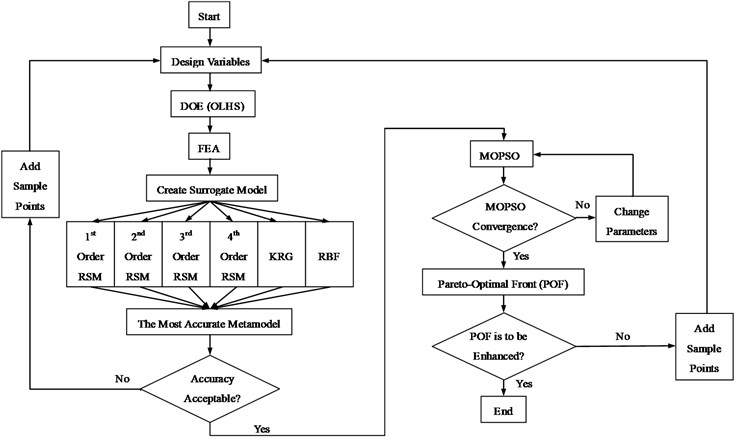It takes a long time and a lot of hurdles to obtain FEA results used in the process of searching optimal mathematical solution especially for evolutionary algorithm often employed in multi-objective and multi-constraint optimization problem that involves large number of iterations. As an alternative, surrogate model is an effective way to formulate the relationship between the design variables and responses mathematically, which will also reduce the cost of computation. In general, for different problems, the precision of different kinds of surrogate models are not the same. In this investigation, three different surrogate models, PRS, KRG, and RBF are considered. Among these surrogates, the most accurate surrogate will be picked up to perform the multi-objective optimization design. To construct high accuracy surrogate, of many different DOE methods available, OLHS approach is selected to generate the training points in the design space here. In order to capture the global maximum dynamic stress in transient shock with nonlinear responses, 100 training points are generated first in this study [22, 24, 25, 33]. It should be noted that if the accuracy of metamodels based on the initial OLHS is unacceptable, the optimization procedure should be returned to the DOE step and more sampling points will be generated in addition to the existing sample points in which a max-min distance criterion is used for inserting the new points to the existing sample space . Given the existing sample set ${X}_{p}$ ($s$ samples), a new sample set ${X}_{c}$ ($t$ samples) will be selected based on the max-min distance approach to maximize the minimum distance between any two sample points in the total sample set ${X}_{A}={X}_{p}{\cup X}_{c}$, i.e.:

14
$\mathrm{m}\mathrm{a}\mathrm{x}\left[{\mathrm{m}\mathrm{i}\mathrm{n}}_{1\le i\le s,1\le j\le s+t}^{{X}_{Ci}\ne {X}_{Aj}}\left(d\left({X}_{Ci},{X}_{Aj}\right)\right)\right],$

where ${X}_{Ci}\in {X}_{C}$ ($i=$ 1,…, $s$), ${X}_{Aj}\in {X}_{A}$ ($j=$1,…, $s+t$).

To obtain the optimal multi-objective design of the battery pack, MOPSO algorithm, which has relatively fast convergence property and well-distributed Pareto frontier, is employed in this paper. More sampling points will also be added based on the max-min distance criterion mentioned above if the Pareto Optimal Frontier needs to be enhanced. For clarification, the proposed optimization procedure is summarized in the flowchart as Fig. 8.

## 4. Results and discussions

### 4.1. Selection and analysis of surrogate models

For the sake of choosing the most accurate and appropriate metamodels for multidiscipline problem in this study, the abovementioned three individual surrogates, namely, PRS (from 1st order polynomial to 4th order polynomial), RBF and KRG are constructed and used to run the optimization. Since the mass of the battery pack follows a linear relationship to the panel thicknesses, the 1st order PRS is adopted to model the weight. To assess the accuracy of these different surrogate schemes modeling the dynamic and transient shock responses of battery pack, 30 new validation points are also generated using the OLHS approach here. Three numerical estimators, namely R-square (R2), relative average absolute error (RAAE) and relative maximum absolute error (RMAE)  are used to measure the accuracy of these metamodels, as given in Eqs. (12). The computational results are summarized in Table. 3.

Table 3Accuracy assessment for different metamodels

 Response Metamodels R2 RAAE RMAE 1st order restrained frequency (Hz) 1st order PSM 0.9476 0.1808 0.6200 2nd order PSM 0.9902 0.0805 0.1938 3rd order PSM 0.9869 0.0926 0.2377 4th order PSM 0.9865 0.0936 0.2406 KRG 0.9795 0.0977 0.5329 RBF 0.9880 0.0751 0.4115 Max. stress under $x$-axis transient shock 1st order PSM 0.6308 0.4780 1.4144 2nd order PSM 0.8335 0.3184 0.9471 3rd order PSM 0.8440 0.3180 0.8068 4th order PSM 0.8156 0.3380 0.9631 KRG 0.7881 0.3322 1.3287 RBF 0.7757 0.4066 1.0686 Max. stress under $y$-axis transient shock 1st order PSM 0.7746 0.3966 1.3593 2nd order PSM 0.8926 0.2656 0.7967 3rd order PSM 0.8732 0.2948 0.6143 4th order PSM 0.8467 0.3258 0.8305 KRG 0.8809 0.2382 1.3066 RBF 0.9109 0.2053 1.2028

For the prediction of restrained basic frequency in this study, similar to , 2nd order RSM is the most accurate and appropriate surrogate model with the R2 value of 0.9902 and RAAE value of 0.0805, which indicates a good performance for overall design space. As for transient acceleration shock analysis, which has relatively high nonlinearity degrees, 3rd order RSM and RBF are the most suitable metamodels for maximum global stress prediction of battery pack under $x$-axis and $y$-axis transient shock, respectively.

### 4.2. Results of MOPSO

Fig. 9 depicts the final Pareto optimal frontier (POF) of the multi-objective optimization for the battery pack, where the settings of MOPSO are list in Table 4.

POF converges adequately after 100 generations. A clear convexity of the curve can be observed, which provides the alternative design space for designers. It is noted that the exploration of the Pareto sets is crucial to fully understand the solution space for the multi-objective optimization problems. The POF provides the designer with a range of optimal solutions for their further decision-making. In all these Pareto plots it is shown that the mass and restrained basic frequency strongly compete with each other, with the range of mass variation from 0.3235-0.3501 ton, alongside with the range of frequency variation from 36.09-31.88 Hz. Obviously, owing to the effect of the restraint of maximum stress response under acceleration shock on the design domain, after optimization, all the values of mass and restrained basic frequency in the Pareto optimal solutions are over and below the initial design, respectively. Specifically, if the designers pay more attention to the weight reduction, the solutions at the bottom right corner should be selected. While if the designers emphasize on restrained basic frequency, the solutions at the top left corner will be considered.

Fig. 9Pareto optimal frontier (POF) and knee point of MOPSO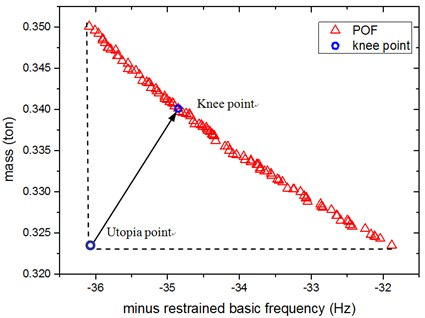Table 4Parameter setting of MOPSO

 Parameters of MOPSO Value Population size 100 External archive size 100 Inertial weight 0.73 Personal learning coefficient 1.50 Global learning coefficient 1.50

Thickness variation range of these eight design variables in Pareto optimal solution set is illustrated from Fig. 10(a) to Fig. 10(h). Apparently, from the eight variables, the thicknesses of the floor are distributed relatively uniform and almost occupied the whole variable space. That is because of the floor has a large surface area compared to other parts, which makes the total battery pack mass very sensitive to this floor thickness change. With the function of battery fixing for fear of its up-and-down movement in the pack, apron set is thinner, and has a size change basically between 0.5 to 1mm, since in this study we do not consider the maximum stress response under $z$-axis acceleration transient shock. Air intake plate, air outlet plate and air outlet chamber are relatively thicker with the size no less than 1.0 mm in order to meet the stress requirement in $x$-axis acceleration shock. For the sake of improving the 1st order restrained natural frequency, cover thickness should not less than 1.0 mm. The eighth design variable, namely, the thickness of the beam set, almost keeps 1.5 mm which is the upper bound of design variables in all Pareto optimal solutions for considering the reinforcement and connection functions of these beams.

Fig. 10Thickness range of eight design variables in Pareto sets after optimization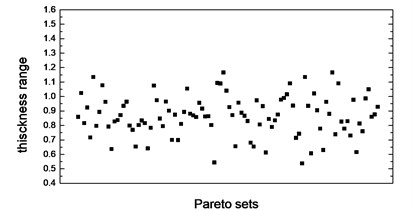a) Thickness range of floor in Pareto sets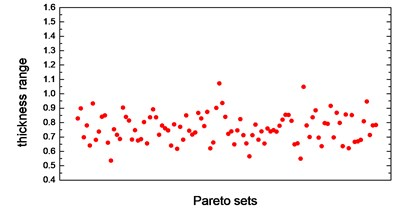b) Thickness range of apron in Pareto sets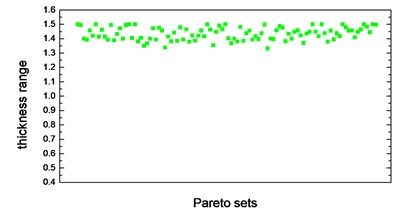c) Thickness range of air intake plate in Pareto sets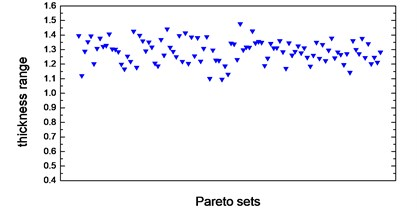d) Thickness range of cover in Pareto sets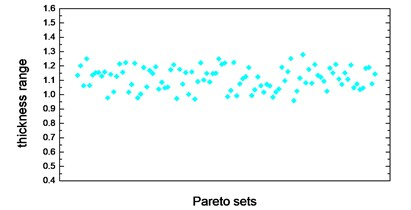e) Thickness range of air outlet plate in Pareto sets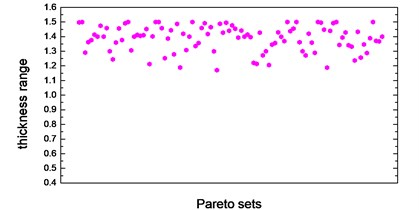f) Thickness range of air outlet chamber in Pareto sets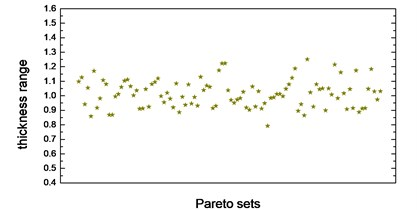g) Thickness range of side wall in Pareto sets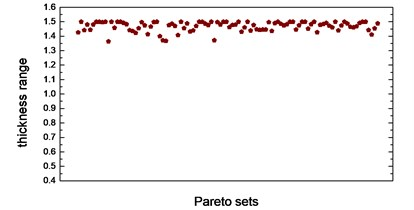h) Thickness range of beam set in Pareto sets

To select the most apposite and the best trade-off optimization scheme, Knee Point, as a normalization to determine the accuracy improvement of Minimum Distance, mathematically given as below, allows us determining a most satisfactory solution from Pareto set :

15
$\mathrm{m}\mathrm{i}\mathrm{n}D=\sqrt{\left({{\sum }_{\tau =1}^{n}\left(\frac{{f}_{c\tau }}{\mathrm{min}\left({f}_{\tau }\left(x\right)\right)}-1\right)}^{2}\right)},$

where $n$ is the number of the objective components, ${f}_{c\tau }$ is the $\tau$th objective value in the $c$th Pareto solution, $D$ is the distance from knee point to an “utopia point”.

Compared with the initial design, it seems that the MOPSO knee point in this study deteriorate both of the two objectives (increase the mass and reduce the restrained basic frequency), which seems that our optimization study is meaningless. Actually, as above-mentioned, considering the restraint of maximum stress response under $x$-axis and $y$-axis acceleration shock, it is inevitably to increase the total mass of the battery pack to increase its strength, but simultaneously cause the basic frequency reduction. Our optimization study is just based on this situation. As shown in Fig. 9, frequency variation range in Pareto optimal solution is not much, just between 31.88-36.09 Hz, and the lower bound 31.88 Hz is over 30 Hz which is satisfactory for automotive devices . Taking account of the lightest weight optimization scheme corresponding to restrained basic frequency is 31.88 Hz, this scheme is also meaningful and useful which should be selected as an alternative alongside with the Knee Point. The validation results of the two alternative optimum schemes using FEA, and their comparison with baseline are summarized in Table 5. There is small difference between the metamodel and FEA results as shown in Table 5. Both of the two schemes satisfy all these two stress response constraints presented. Nevertheless, it is noted that the Max. stress response under y-axis acceleration shock of Knee Point scheme has been pushed to the boundary of the constraint after optimization, which could lead to an unreliable solution if any perturbation of design variables presents.

Table 5Comparison between baseline and MOO optimal design

 Description Baseline Optimization scheme 1 (knee point) Optimization scheme 2 (minimum mass) Metamodel FEA Difference Metamodel FEA Difference Objectives Mass 0.3211 ton 0.3402 ton 0.3404 ton –0.059 % 0.3231 ton 0.3253 ton –0.68 % Restrained basic frequency 38 Hz 35.0 Hz 34.8 Hz 0.6 % 31.9 Hz 32.2 Hz –0.93 % Constraints Max. stress under $x$-axis shock 402.8 MPa 300.7 MPa 311.9 MPa –3.6 % 364.0 MPa 344.5 MPa 5.7 % Max. stress under $y$-axis shock 422.9 MPa 380.0 MPa 367.5 MPa 3.4 % 378.2 MPa 371.5 MPa 1.8 % Variables(thickness) Floor 0.6 mm 1.14 mm – – 0.56 mm – – Apron 1.0 mm 0.94 mm – – 0.63 mm – – Air intake plate 0.8 mm 1.49 mm – – 1.39 mm – Cover 0.8 mm 1.23 mm – – 1.38 mm – – Air outlet plate 0.8 mm 1.17 mm – – 1.02 mm – – Air outlet chamber 1.0 mm 1.50 mm – – 1.20 mm – – Side wall 0.8 mm 1.20 mm – – 0.88 mm – – Beam set 1.2 mm 1.50 mm – – 1.43 mm – –

## 5. Conclusions

To carry and protect the lithium-ion power battery set equipped on electric vehicles, a battery pack is designed in this work. With the simplification of battery model as appropriate, FEA results of the baseline design show that the maximum von Mises stress responses under battery pack $x$-axis and y-axis transient acceleration shock condition are both above the tensile limit of material. In addition to stress response, taking into account the mass and restrained basic frequency, a multi-objective and multi-constraints size optimization problem is necessary to carry out herein. The OLHS technique is first used to sample the points over the design space. Then, based on the limited sampling data obtained, different surrogate schemes, including PRS, KRG, and RBF, are established to relate the total mass, restrained basic frequency and global Max. stress in $x$-axis and $y$-axis shock cases to the design variables (thickness of panels and beams). It is found that 2nd order RSM, 3rd order RSM and RBF are the most accurate and appropriate metamodels for restrained basic frequency, global Max. stress under $x$-axis and $y$-axis shock condition, respectively. To minimize the total mass and simultaneously maximize the restrained basic frequency with the constraints of stress response, a multi-objective PSO (MOPSO) programme is implemented in this work. Owing to the restriction of global maximum stress response constraint, all of the optimal solutions in Pareto optimal frontier have heavier weight and lower frequency compared with baseline design. Two optimal schemes, namely, Knee Point and lightest weight, satisfied both of the stress constraint conditions, show great consistency with FEA results and can be selected as alternative improved schemes. These two battery packs will be fabricated and tested in our next work.

#### Cited by

Revealing the influence of solution and pre-deformation treatment on the corrosion resistance of Y-modified AZ91 magnesium alloy for lithium-ion battery shell
Metamodel-Based Multi-Objective Reliable Optimization for Front Structure of Electric Vehicle
2018 IEEE Vehicle Power and Propulsion Conference (VPPC)

17 January 2016
Accepted
30 May 2016
Published
30 June 2016
SUBJECTS
Vibration in transportation engineering
Keywords
battery pack
surrogate-based optimization
restrained basic frequency analysis
acceleration shock response
multi-objective PSO (MOPSO)
multidisciplinary optimization
multiple surrogate models
Acknowledgements

This work is supported by The National Natural Science Foundation of China (51205023, 51275040) and The National Key Technology R&D Program of China (2014BAG02B02).